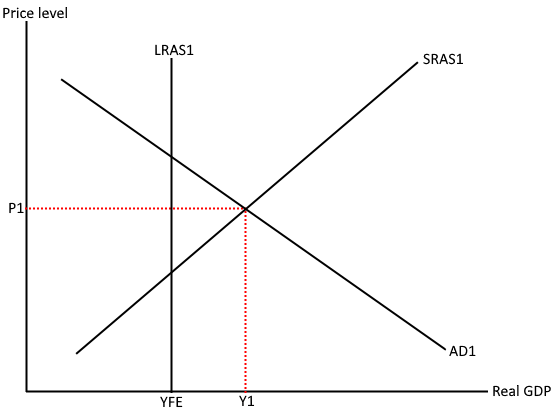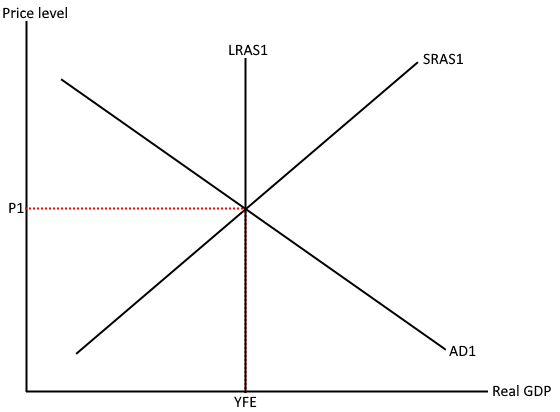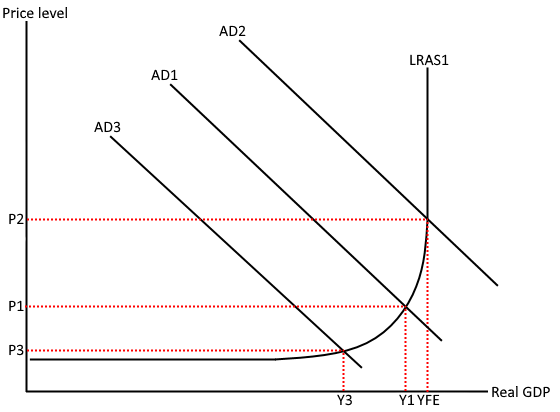# Equilibrium levels of real national output

A) The concept of equilibrium real national output

Equilibrium real national output occurs at the point where AS is equal to AD. However, due to the fact that there are different economic models of AD/AS, there are also different ways of showing macroeconomic equilibrium. This is especially the case for the classical model as it differentiates between long-run AS and short run AS. This means that there is a long run equilibrium point as well as a short run equilibrium point.

ClassicalThe short run equilibrium occurs at the point where AD is equal to SRAS, but not LRAS. As shown from the diagram above, the short run equilibrium point occurs where SRAS1 =AD1. This gives a price level of P1 and output of Y1. The short run equilibrium is able to go past the full employment level. This is due to the fact that full employment is the maximum level of output that an economy can achieve using all factors of production at sustainable levels. Therefore the economy is able to go past this point through the unsustainable use of factors of production e.g. paying workers overtime.The short term equilibrium point can also occur before the full employment point. In this example the economy is operating at the point where AD1 = AS1, giving a price level of P1 and output of Y1. The difference between Y1 and YFE is known as a negative output gap.In the long run, wages become variable and therefore the economy adjusts to a point of full employment through the reduction in wage costs for firms. Long run equilibrium is shown in this diagram as LRAS=SRAS=AD. The classical theory states that an economy will always be at full employment in the long run.

Keynesian

Unlike the classical model, the Keynesian model does not differentiate between short run and long run. Instead of assuming wages will become variable in the long run, Keynesian believed that the long run equilibrium point could be at any point where AD=LRAS. Therefore, active policy was needed in order to shift AD to the right and move the economy closer to the full employment level (YFE).As shown by the diagram, although the economy is currently operating far from full employment, the point where AD1=LRAS1 is the long run equilibrium. This means that without any form of expansionary policy in place, the output gap of Y1 to YFE will exist in the long run.

B) The use of AD/AS diagrams to show how shifts in AD or AS cause changes in the equilibrium price level and real national output

Shifts in AD – Classical modelAn increase in AD (AD1 to AD2) causes an increase in real GDP (Y1-YFE) moving the economy to a point of full employment (YFE). The bigger the increase in AD, the closer the shift will take the economy to the point of full employment. This increase in AD causes the price level to increase from P1 to P2 as a result of the reduction in spare capacity putting more pressure on existing factors of production. On the other hand, a decrease in AD causes a reduction in real GDP (Y1 to Y3) and a decrease in the price level (P1 to P3).

Shifts in AS – Classical modelA decrease in costs of production causes SRAS to increase (SRAS1 to SRAS2) resulting in an increase in real GDP (Y1 to Y2) and a decrease in the price level (P1 to P2). This is due to the fall in the price of key inputs allowing firms to sell their goods/services at a lower price. On the other hand, a decrease in SRAS (SRAS1 to SRAS3) causes a decrease in real GDP (Y1 to Y3) and an increase in the price level (P1 to P3). This is because firms pass some of the increased costs of production onto consumers in the form of higher prices (cost push inflation).

Shifts in LRAS – Classical modelAn increase in LRAS causes an increase in Real GDP and a decrease in cost push inflation (P1-P2). Conversely, a decrease in LRAS (LRAS1 to LRAS3) will cause a decrease in Real GDP (Y1 to Y2) and an increase in the price level (P1 to P2).

Shifts in AD – Keynesian modelAn increase in aggregate demand (AD1 to AD2) will cause an increase in real GDP (Y1 to YFE) and an increase in the price level (P1 to P2).